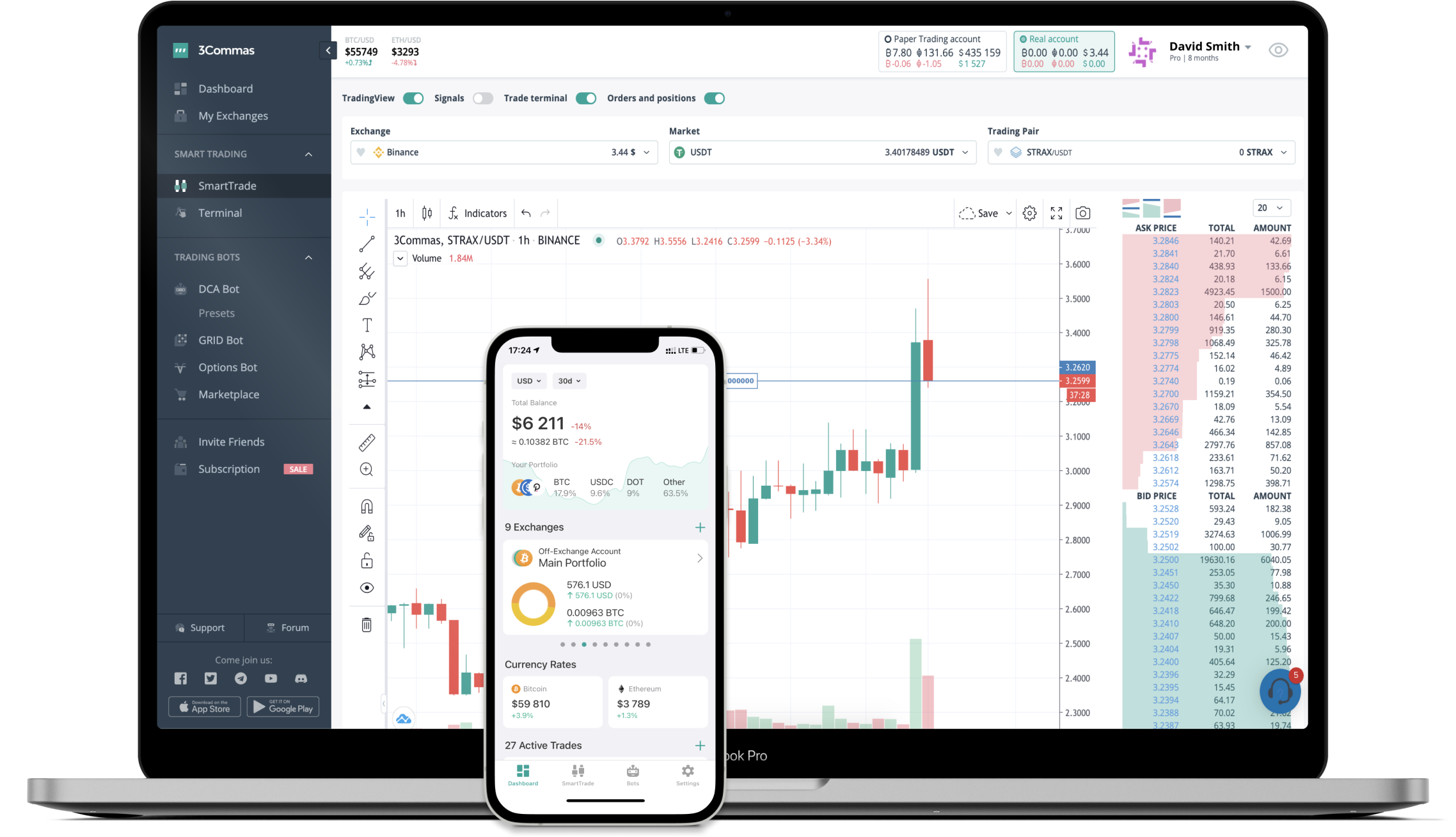# DELTA to KRW Converter

Delta Financial
PriceKRW 1,550.82+0.58%
VolumeKRW 129,083
How it work

The 3Commas currency calculator allows you to convert a currency from Delta Financial (DELTA) to South Korean Won (KRW) in just a few clicks at live exchange rates.

Simply enter the amount of Delta Financial you wish to convert to KRW and the conversion amount automatically populates. You can also use our Prices Calculator Table to calculate how much your currency is worth in other denominations, i.e. .1 DELTA, .5 DELTA, 1 DELTA, 5 DELTA, or even 10 DELTA.

## DELTA to KRW Exchange Calculator

The chart displays Delta Financial price changes in KRW.

The ultimate tools for crypto traders to maximize trading profits while minimizing risk and loss.## Latest DELTA to KRW price calculator

Delta Financial market price is updated every three minutes and is automatically displayed in KRW. Below are the most popular denominations to convert to KRW.

Delta Financial
South Korean Won
15.51
KRW
155.08
KRW
1550.82
KRW
3101.64
KRW
4652.46
KRW
7754.10
KRW
15508.20
KRW
38770.50
KRW
77541.00
KRW
155082.00
KRW
387705.00
KRW
775410.00
KRW
1550820.00
KRW
3877050.00
KRW
South Korean Won
Delta Financial
0.00000645
DELTA
0.00006448
DELTA
0.00064482
DELTA
0.00128964
DELTA
0.00193446
DELTA
0.00322410
DELTA
0.00644820
DELTA
0.01612050
DELTA
0.03224101
DELTA
0.06448202
DELTA
0.16120504
DELTA
0.32241008
DELTA
0.64482016
DELTA
1.61205040
DELTA

## FAQ

### How much is 1 Delta Financial in South Korean Won?

Delta Financial price in KRW is constantly changing.

At this moment, 1 Delta Financial equals 1550.82 KRW

### How do I calculate the conversion from DELTA to KRW?

The 3Commas Delta Financial Calculator allows you to easily calculate the conversion price of DELTA to KRW by simply entering the amount of Delta Financial in the corresponding field and will automatically convert the value in South Korean Won (KRW).

You can also use our Delta Financial price table above to check the latest Delta Financial price in major fiat and crypto currencies.

### How can I convert Delta Financial to KRW?

The most common way of converting DELTA to KRW is by using a Crypto Exchange or a P2P (person-to-person) exchange platform like LocalBitcoins, etc.